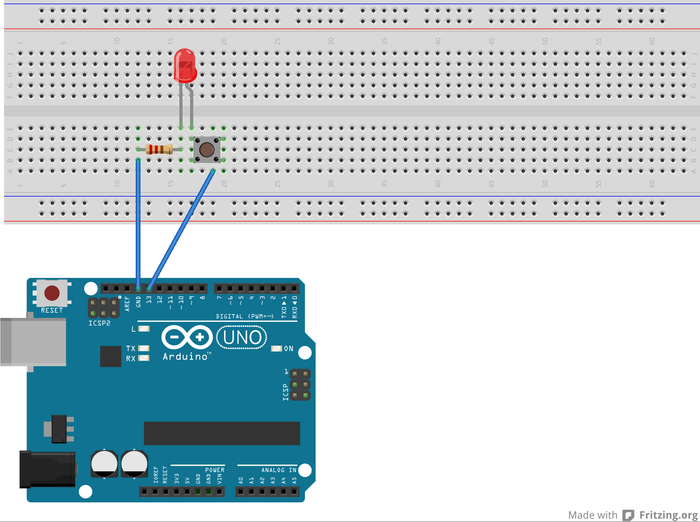# Arduino Introduction

Arduino is a open source hardware plataform for protoyping, makes easy for a non electronic engineer builds interative objects. This post is an introduction, most of the information has been taken from arduino website. Some of the images has been create with Fritzing

### IDE Instalation

Arduino IDE is multiplatform, check this link to find the instructions for your plataform http://arduino.cc/en/Main/Software

### Blinkin a Led

The most basic example to test the arduino is blinking a led, a complete tutorial about this can be found a this link

#### The circuit

attach a 220-ohm resistor(red one end and gold another end) to pin 13. Then attach the long leg of an LED (the positive leg, called the anode) to the resistor. Attach the short leg (the negative leg, called the cathode) to ground

#### The code

Open the arduino ide and paste the next code

const int LED = 13; // LED connected to
// digital pin 13

void setup()
{
pinMode(LED, OUTPUT);    // sets the digital
// pin as output
}

/**1. Turns on the led
2. Wait one second
3. Tuns off the led
4. Wait one second
**/
void loop()
{
digitalWrite(LED, HIGH);
delay(1000);
digitalWrite(LED, LOW);
delay(1000);
}


Compile and upload the code to the arduino board.

### Saying hello world in morse code

Morse code has been used for years to communicate visually through great distances. Let's try To improve the blink skethc to say hello world, this in morse code is:

• H: ....
• E: .
• L: .---
• O: ---
• W: .--
• R: .-.
• D: -..
.... . .--- .--- ---  .-- --- .-. .--- -..

For every dash the led will be on by 1 second, by every point for 0.5 second and between words willbe a 1.5 second delay

#### The circuit

Is the same circuit that the previous one, but this use the protoboard:

#### The code

    const int LED = 13; // LED connected to
// digital pin 13

void setup()
{
pinMode(LED, OUTPUT);    // sets the digital
// pin as output
}

void point(){
digitalWrite(LED, HIGH);
delay(800);
digitalWrite(LED, LOW);
delay(200);
}

void dash(){
digitalWrite(LED, HIGH);
delay(1600);
digitalWrite(LED, LOW);
delay(200);
}

void space(){
delay(500);
}

/**print "hello world" in morse code
**/
void sayHello(){
point();
point();
point();
point();
space();
point();
space();
point();
dash();
dash();
dash();
space();
point();
dash();
dash();
dash();
space();
dash();
dash();
dash();
space();
space();
space();
point();
dash();
dash();
space();
dash();
dash();
dash();
space();
point();
dash();
point();
space();
point();
dash();
dash();
dash();
space();
dash();
point();
point();
space();
space();
space();

}

void loop()
{
sayHello();
}



#### Putting a Swith in the circuitSource code of this examples here